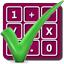#### Arithmetic of Computers

#### from Tenscope Limited

By using this site, you are accepting "session" cookies, as set out in the site's Privacy Policy
Cookies are also used to remember which page of the book you last viewed, so that when you revisit the site you automatically return to the last page you visited.

### Lesson 7

#### Page 221

The conversion of octal numbers to decimal numbers is accomplished, as we have seen, by writing the number in expanded form and performing the arithmetic operations.
The opposite conversion - from decimal to octal - is accomplished by an elegant method in which the remainders from division operations and the integral parts of products play leading roles.
You recall that before you knew about decimal fractions, when you had to work a division problem that did not “come out even.” you simply noted the remainder instead of carrying the quotient out to a decimal fraction. For example, 25 divided by 8 results in a quotient of 3, with a remainder of 1. (The following calculations are all done with decimal numbers.)
3 8)25 24 1  = remainder
In the division of 46 by 4, what is the remainder?